# Chapter 6 dplyr: Manipulating Data Frames

To work with data, we need a place to store it in R. Our default setting is to store data in data frames in a tidy format$$^{**}$$. When we work with properly formatted data frames, $$^{**}$$ Data will not always be stored in a way that is amenable to analysis. Typically, we will get our data into a tidy format - such that each row represents an observation and each column represents an attribute or property of that observation each data frame can be thought of as a collection of observations with each observation in its own row and each recorded variable (e.g. measurement) represented in a column; a generic dataframe with $$M$$ rows and $$N+1$$ columns (i.e. $$N$$ variables plus an id column) is shown in Table 6.1.

Table 6.1: Think of a data frame as consisting of rows of observations and columns of variables.

observationID var1 var2 var… varN
1 xx xx xx xx
2 xx xx xx xx
$$\vdots$$ $$\vdots$$ $$\vdots$$ $$\vdots$$ $$\vdots$$
M xx xx xx xx

We can look at a subset of the built-in mtcars dataset as a more tangible example of a tidy dataframe - from the console, type mtcars and press <ENTER>:

carID mpg cyl disp hp drat wt qsec vs am gear carb
Mazda RX4 21.0 6 160.0 110 3.90 2.620 16.46 0 1 4 4
Honda Civic 30.4 4 75.7 52 4.93 1.615 18.52 1 1 4 2
Camaro Z28 13.3 8 350.0 245 3.73 3.840 15.41 0 0 3 4
Volvo 142E 21.4 4 121.0 109 4.11 2.780 18.60 1 1 4 2

Each row represents a particular car and the recorded data associated with each car is organized by column. Note that an ID column is also just an attribute of that observation.

There are infinite ways data can be non-tidy and thus, harder to work with. Two non-tidy examples, assuming the observational unit is still just one car, might look like this:

carID measure value
Mazda RX4 mpg 21.0
Honda Civic mpg 30.4
Camaro Z28 mpg 13.3
Volvo 142E mpg 21.4
Mazda RX4 cyl 6.0
Honda Civic cyl 4.0
Camaro Z28 cyl 8.0
Volvo 142E cyl 4.0

where one particular observational unit (e.g. Mazda RX4) is on multiple rows. Or alternatively, this is also non-tidy:

carID mpg eng:cyl_disp hp drat wt qsec vs am gear carb
Mazda RX4 21.0 6 - 160 110 3.90 2.620 16.46 0 1 4 4
Honda Civic 30.4 4 - 75.7 52 4.93 1.615 18.52 1 1 4 2
Camaro Z28 13.3 8 - 350 245 3.73 3.840 15.41 0 0 3 4
Volvo 142E 21.4 4 - 121 109 4.11 2.780 18.60 1 1 4 2

where multiple variables (i.e. cyl and disp) might be stored together in one column. See (https://tidyr.tidyverse.org/articles/tidy-data.html) for further examples of messy data.

## 6.1 Tibbles

While R’s data frame object has been the long running standard placeholder for data, we will often convert data frame’s into tibble objects. The as_tibble function from the dplyr package does this conversion for us:

Remember, in order to load a package into an R session using library(packageName), you must have the package installed on your system. dplyr was installed in previous chapters, so this code should work without error.

# make the dplyr package and its function available
# in the current R session.
library(dplyr)

## convert the built in mtcars dataframe to a tibble
as_tibble(mtcars)
## # A tibble: 32 x 11
##      mpg   cyl  disp    hp  drat    wt  qsec    vs    am  gear  carb
##    <dbl> <dbl> <dbl> <dbl> <dbl> <dbl> <dbl> <dbl> <dbl> <dbl> <dbl>
##  1  21       6  160    110  3.9   2.62  16.5     0     1     4     4
##  2  21       6  160    110  3.9   2.88  17.0     0     1     4     4
##  3  22.8     4  108     93  3.85  2.32  18.6     1     1     4     1
##  4  21.4     6  258    110  3.08  3.22  19.4     1     0     3     1
##  5  18.7     8  360    175  3.15  3.44  17.0     0     0     3     2
##  6  18.1     6  225    105  2.76  3.46  20.2     1     0     3     1
##  7  14.3     8  360    245  3.21  3.57  15.8     0     0     3     4
##  8  24.4     4  147.    62  3.69  3.19  20       1     0     4     2
##  9  22.8     4  141.    95  3.92  3.15  22.9     1     0     4     2
## 10  19.2     6  168.   123  3.92  3.44  18.3     1     0     4     4
## # ... with 22 more rows

The main advantage of a tibble is that when it is printed out, it will not try to print all the data. By default, tibble’s show the first 10 rows of data and as many columns as will fit on your screen. Once we start working with datasets that have tens of thousands of rows and dozens of columns, you will then appreciate the tibble. In this book, we will use the terms tibble and data frame synonymously because they differ only very slightly in their behavior. Most of the time, Internet resources will almost exclusively refer to data frames as the object for data storage - the tibble terminology is far less ubiquitous - so use data frame as your google search term.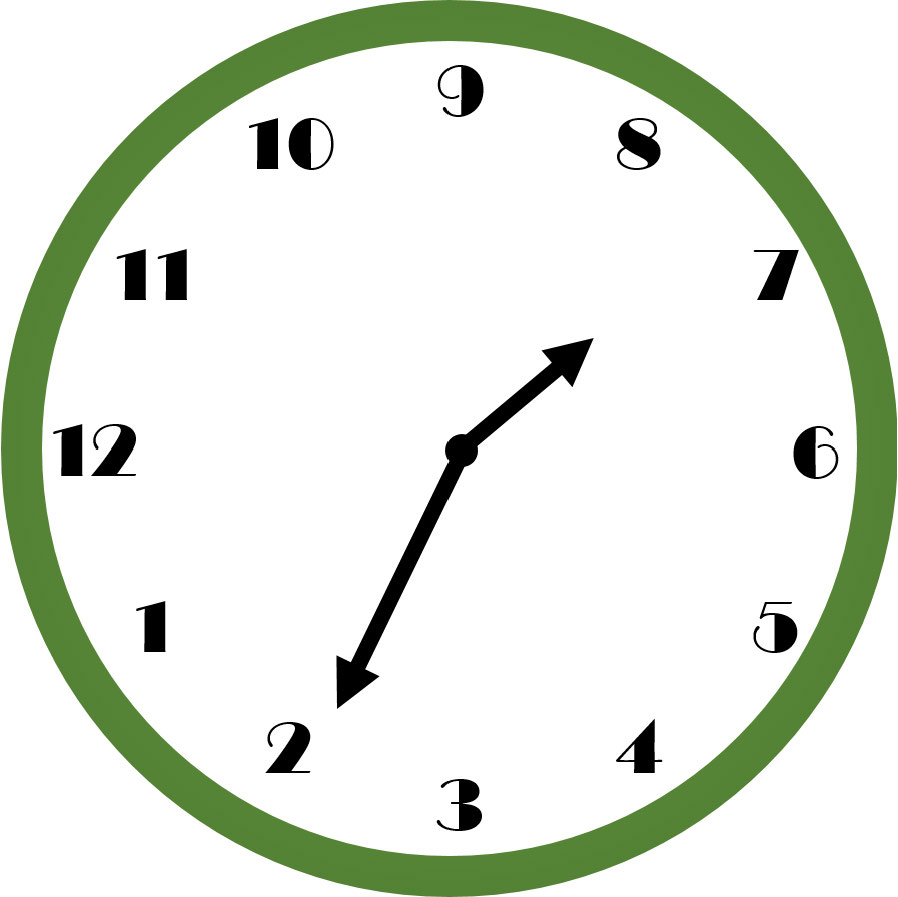Figure 6.1: What time does this clock read? A non-standardized design increases the amount of thinking time required to get the answer. After significant deliberation, hopefully you see that the time is 7:11.

Data manipulation is not a natural human task - there is definitely some mental gymnastics required. To simplify the cognitive load, we will adopt a standard way of thinking about data manipulation. These standards will reduce the cognitive load, i.e. thinking time, required of your brain as you get data into more useful forms. An example standard that you might take for granted is that used by clock makers; see the example in Figure 6.1 and you will quickly realize, by example, how important standards can be in aiding your thinking (example from Norman 2013Norman, Don. 2013. The Design of Everyday Things: Revised and Expanded Edition. Basic Books (AZ).).

When it comes to data manipulation, the standards we will learn in this chapter are implemented in the dplyr package. The dplyr package is part of the tidyverse (https://www.tidyverse.org/) ecosystem of packages. These packages are all designed to reduce cognitive load through a set of well-thought out standards which share an underlying design philosophy and structure. The dplyr package simplifies our thought process in regards to data manipulation by reducing our possible operations to five main verbs and one adverb. It then facilitates the chaining of these operations to accomplish even the most difficult of data manipulation tasks. The five main verbs are: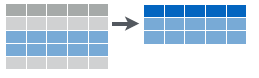Figure 6.2: Filtering data to get a subset of rows.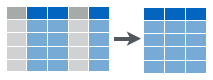Figure 6.3: Selecting data to get a subset of columns.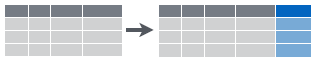Figure 6.4: Create new columns that are functions of existing columns using mutate.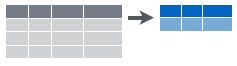Figure 6.5: Collapsing data into summary metrics using summarize.

1. filter(): select subset of rows (i.e. observations). See Figure 6.2.
2. arrange(): reorder rows
3. select(): select subset of columns (i.e. variables). See Figure 6.3.
4. mutate(): create new columns that are functions of existing columns. See Figure 6.4.
5. summarize(): collapse data into a single row. See Figure 6.5.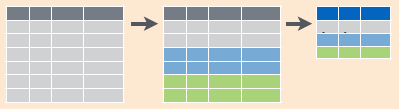Figure 6.6: Collapsing data into summary metrics using group_by() with summarize().

These verbs are useful on their own, but they become really powerful when you apply them to groups of observations within a dataset using the group_by() function (i.e. the one adverb). It breaks down a dataset into specified groups of rows so that subsequent verbs may act on grouped rows and not just the entire dataset.

Grouping affects the verbs as follows:

• arrange() orders first by the grouping variables and then by the variables of arrange
• summarize() is the most powerful to combine with grouping. You use summarize with aggregate functions, which take a vector of values and return a single number. See Figure 6.6. There are many useful examples of aggregate functions in base R like min(), max(), mean(), sum(), sd(), median(), and IQR(). dplyr provides a handful of others:
• n(): the number of observations in the current group
• n_distinct(): the number of unique values in x.
• first(x), last(x) and nth(x, n): these work similarly to x, x[length(x)], and x[n], but give you more control over the result if the value is missing.

Learning dplyr is perhaps easiest by example. Below, we will provide code for you to follow the vignette that accompanies the dplyr package.$$^{**}$$ see https://dplyr.tidyverse.org/ if interested in more detail We’ll start with the built in nycflights13 data frame. This dataset contains all 336,776 flights that departed from New York City in 2013 (see link in margin).

## Uncomment the install line if the package has not
## been installed on your computer.
#install.packages("nycflights13", dependencies = TRUE)
#install.packages("dplyr", dependencies = TRUE)
library(nycflights13)
library(dplyr)

## Show the dataframe in the RStudio envirnoment
flights = flights

## just fyi, there are lots of datasets already in R
data()

## 6.3 filter() Rows

filter allows you to select a subset of rows in a data frame. The first argument is the name of the data frame. The second and subsequent arguments are the expressions that filter the data frame:

For example, we can select all flights on January 1st with:Take note of the double equal sign $$==$$ which is used for logical comparison. The single equal sign $$=$$ is only used for assignment purposes.

filter(flights, day == 1, month == 1)
## # A tibble: 842 x 19
##     year month   day dep_time sched_dep_time dep_delay arr_time sched_arr_time
##    <int> <int> <int>    <int>          <int>     <dbl>    <int>          <int>
##  1  2013     1     1      517            515         2      830            819
##  2  2013     1     1      533            529         4      850            830
##  3  2013     1     1      542            540         2      923            850
##  4  2013     1     1      544            545        -1     1004           1022
##  5  2013     1     1      554            600        -6      812            837
##  6  2013     1     1      554            558        -4      740            728
##  7  2013     1     1      555            600        -5      913            854
##  8  2013     1     1      557            600        -3      709            723
##  9  2013     1     1      557            600        -3      838            846
## 10  2013     1     1      558            600        -2      753            745
## # ... with 832 more rows, and 11 more variables: arr_delay <dbl>,
## #   carrier <chr>, flight <int>, tailnum <chr>, origin <chr>, dest <chr>,
## #   air_time <dbl>, distance <dbl>, hour <dbl>, minute <dbl>, time_hour <dttm>
Exercise 6.1 Create a new data frame called that finds all flights where the carrier was United Airlines.

## 6.4 arrange() Rows

arrange() works similarly to filter() except that instead of filtering or selecting rows, it reorders them. It takes a data frame, and a set of column names (or more complicated expressions) to order by. If you provide more than one column name, each additional column will be used to break ties in the values of preceding columns.

arrange(flights, year, month, day)
## # A tibble: 336,776 x 19
##     year month   day dep_time sched_dep_time dep_delay arr_time sched_arr_time
##    <int> <int> <int>    <int>          <int>     <dbl>    <int>          <int>
##  1  2013     1     1      517            515         2      830            819
##  2  2013     1     1      533            529         4      850            830
##  3  2013     1     1      542            540         2      923            850
##  4  2013     1     1      544            545        -1     1004           1022
##  5  2013     1     1      554            600        -6      812            837
##  6  2013     1     1      554            558        -4      740            728
##  7  2013     1     1      555            600        -5      913            854
##  8  2013     1     1      557            600        -3      709            723
##  9  2013     1     1      557            600        -3      838            846
## 10  2013     1     1      558            600        -2      753            745
## # ... with 336,766 more rows, and 11 more variables: arr_delay <dbl>,
## #   carrier <chr>, flight <int>, tailnum <chr>, origin <chr>, dest <chr>,
## #   air_time <dbl>, distance <dbl>, hour <dbl>, minute <dbl>, time_hour <dttm>

Use desc() to order a column in descending order

arrange(flights, desc(arr_delay))
## # A tibble: 336,776 x 19
##     year month   day dep_time sched_dep_time dep_delay arr_time sched_arr_time
##    <int> <int> <int>    <int>          <int>     <dbl>    <int>          <int>
##  1  2013     1     9      641            900      1301     1242           1530
##  2  2013     6    15     1432           1935      1137     1607           2120
##  3  2013     1    10     1121           1635      1126     1239           1810
##  4  2013     9    20     1139           1845      1014     1457           2210
##  5  2013     7    22      845           1600      1005     1044           1815
##  6  2013     4    10     1100           1900       960     1342           2211
##  7  2013     3    17     2321            810       911      135           1020
##  8  2013     7    22     2257            759       898      121           1026
##  9  2013    12     5      756           1700       896     1058           2020
## 10  2013     5     3     1133           2055       878     1250           2215
## # ... with 336,766 more rows, and 11 more variables: arr_delay <dbl>,
## #   carrier <chr>, flight <int>, tailnum <chr>, origin <chr>, dest <chr>,
## #   air_time <dbl>, distance <dbl>, hour <dbl>, minute <dbl>, time_hour <dttm>

## 6.5 select() Columns

Often you work with large datasets with many columns, but only a few are actually of interest to you. select() allows you to rapidly zoom in on a useful subset of columns:

# Select columns by name
select(flights, year, month, day)
## # A tibble: 336,776 x 3
##     year month   day
##    <int> <int> <int>
##  1  2013     1     1
##  2  2013     1     1
##  3  2013     1     1
##  4  2013     1     1
##  5  2013     1     1
##  6  2013     1     1
##  7  2013     1     1
##  8  2013     1     1
##  9  2013     1     1
## 10  2013     1     1
## # ... with 336,766 more rows
# Select all columns between year and day (inclusive)
select(flights, year:day)
## # A tibble: 336,776 x 3
##     year month   day
##    <int> <int> <int>
##  1  2013     1     1
##  2  2013     1     1
##  3  2013     1     1
##  4  2013     1     1
##  5  2013     1     1
##  6  2013     1     1
##  7  2013     1     1
##  8  2013     1     1
##  9  2013     1     1
## 10  2013     1     1
## # ... with 336,766 more rows
# Select all columns except those from year to day (inclusive)
select(flights, -(year:day))
## # A tibble: 336,776 x 16
##    dep_time sched_dep_time dep_delay arr_time sched_arr_time arr_delay carrier
##       <int>          <int>     <dbl>    <int>          <int>     <dbl> <chr>
##  1      517            515         2      830            819        11 UA
##  2      533            529         4      850            830        20 UA
##  3      542            540         2      923            850        33 AA
##  4      544            545        -1     1004           1022       -18 B6
##  5      554            600        -6      812            837       -25 DL
##  6      554            558        -4      740            728        12 UA
##  7      555            600        -5      913            854        19 B6
##  8      557            600        -3      709            723       -14 EV
##  9      557            600        -3      838            846        -8 B6
## 10      558            600        -2      753            745         8 AA
## # ... with 336,766 more rows, and 9 more variables: flight <int>,
## #   tailnum <chr>, origin <chr>, dest <chr>, air_time <dbl>, distance <dbl>,
## #   hour <dbl>, minute <dbl>, time_hour <dttm>

A common use of select() is to pair it with the distinct() verb (a shortcut variant for the filter() verb). This combination returns the unique rows of a smaller data frame. Here we use select() to get just one column (tailNum) and then distinct() returns the unique tail numbers of all the airplanes in the dataset:

distinct(select(flights, tailnum))
## # A tibble: 4,044 x 1
##    tailnum
##    <chr>
##  1 N14228
##  2 N24211
##  3 N619AA
##  4 N804JB
##  5 N668DN
##  6 N39463
##  7 N516JB
##  8 N829AS
##  9 N593JB
## 10 N3ALAA
## # ... with 4,034 more rows
Exercise 6.2 Create a new data frame called routes that consists of two columns which contain all combinations of flight origin and flight destination in the original dataset. How many unique routes are there?

## 6.6 Add New Columns with Mutate()

Besides selecting sets of existing columns, it’s often useful to add new columns that are functions of existing columns. This is the job of mutate():

flightSpeedDF = select(flights, distance, air_time)
mutate(flightSpeedDF,
speed = distance / air_time * 60)
## # A tibble: 336,776 x 3
##    distance air_time speed
##       <dbl>    <dbl> <dbl>
##  1     1400      227  370.
##  2     1416      227  374.
##  3     1089      160  408.
##  4     1576      183  517.
##  5      762      116  394.
##  6      719      150  288.
##  7     1065      158  404.
##  8      229       53  259.
##  9      944      140  405.
## 10      733      138  319.
## # ... with 336,766 more rows

The second argument to the mutate() function (e.g. speed = distance / air_time * 60) defines a new column called speed and it is a function of the existing columns distance and air.

## 6.7 summarize() Values

The last verb is summarize(). It collapses a data frame into a single row by aggregating a column of data. Below, the dep_delay column is summarized using the mean() function:

summarize(flights,
delay = mean(dep_delay, na.rm = TRUE))
## # A tibble: 1 x 1
##   delay
##   <dbl>
## 1  12.6

So instead of seeing a data frame with 336,776 rows, we collapse all those departure delays into a single average number (i.e. 12.6 minutes).

## 6.8 Grouped Operations with group_by()

These five functions provide the basis of a language of data manipulation. At the most basic level, you can only alter a tidy data frame in five useful ways: you can reorder the rows (arrange()), filter the rows (filter()), pick columns of interest (select()), add new columns (mutate()) that are functions of existing variables, or collapse (summarize()) many rows into a summary. The power of the language comes from your ability to combine the five functions, but before we do that - let’s discuss one more function group_by().

The group_by() function does not change the output of a data frame. But it does change how arrange(), mutate(), and summarize() behave. Each of them will act within the unique values of specified grouping variable(s) as if the rows of the data frame were separated into groups before the other verb is called. This is easiest to see by example via the powerful data manipulation enabled by combining group_by() and summarize() functions. The summarize operation will collapse each group of data instead of the entire dataset into a single row. For example, we could use these to find the number of planes and the number of flights that go to each possible destination:

## create a new dataframe that is organized by groups
destinations = group_by(flights, dest)
## summarize the rows of the grouped data frame
destDF = summarize(destinations,
planes = n_distinct(tailnum), # unique planes
flights = n() # number of flights
)
destDF
## # A tibble: 105 x 3
##    dest  planes flights
##    <chr>  <int>   <int>
##  1 ABQ      108     254
##  2 ACK       58     265
##  3 ALB      172     439
##  4 ANC        6       8
##  5 ATL     1180   17215
##  6 AUS      993    2439
##  7 AVL      159     275
##  8 BDL      186     443
##  9 BGR       46     375
## 10 BHM       45     297
## # ... with 95 more rows
Exercise 6.3 Create a new data frame called sortDestDF that orders (i.e. arranges) the destDF dataframe in descending order of popularity (i.e. number of flights from NYC to that destination) to discover the most popular places people from New York City fly to.

## 6.9 Commonalities

You may have noticed that the function syntax for all these verbs is very similar:

• The first argument is a data frame (e.g. flights or flightsDF).
• The subsequent arguments describe what to do with the data frame. Notice that you can refer to columns in the data frame directly without using $. Hence, you do NOT have to write something like flights$dep_delay in the above summarize() function.
• The resulting output is a new data frame.

As is shown in the next section, these properties make it easy to chain together multiple simple steps to achieve a complex result.

## 6.10 Chaining with %>%

In the previous examples, we sometimes had to save results to intermediate dataframes and then do subsequent analysis on the newly created dataframe. For example, if using the original flights data frame we wanted to find the destination airports that had the fastest average (mean) flight speed, we could do the following:

# create new data frame (df) with three columns
# extracted from flights data frame
lightSpeedDF = select(flights, distance, air_time, dest)
# create new data frame with additional column
# representing speed
lightSpeedDF2 = mutate(lightSpeedDF,
speed = distance / air_time * 60)
# create new data frame that has hidden groupings
# by destination
lightSpeedDF3 = group_by(lightSpeedDF2, dest)
# create new data frame that summarizes speed for
# each destination group
lightSpeedDF4 = summarize(
lightSpeedDF3,
avgSpeed = mean(speed, na.rm = TRUE))
# print out a sorted data frame - note that the
# below does not create a new data frame
# as there is no assignment operator (i.e. '=')
arrange(lightSpeedDF4, desc(avgSpeed))
## # A tibble: 105 x 2
##    dest  avgSpeed
##    <chr>    <dbl>
##  1 ANC       490.
##  2 BQN       487.
##  3 SJU       486.
##  4 HNL       484.
##  5 PSE       481.
##  6 STT       479.
##  7 LAX       453.
##  8 SAN       451.
##  9 SMF       451.
## 10 LGB       450.
## # ... with 95 more rows

As an example of the chaining operator in mathematical notation, assume $$f(x,y) = 2*x + y^2$$. Then, $$f(1,3) = 2*1 + 3^2 = 11$$. To write this with chaining, we have $$1$$ %>% $$f(3)$$. Notice that $$3$$ %>% $$f(1)$$ yields different results; in this case, $$3$$ %>% $$f(1) = f(3,1) = 7$$.

This becomes challenging code. It creates several data frames that we are not interested in (e.g. lightSpeedDF2) and is difficult to read. Alternatively, we can leverage the chaining operator, %>%. This operator inserts an R object as the first argument of a function. In mathematical terms, $$x$$ %>% $$f(y)$$ is interpreted as $$f(x,y)$$ - the $$x$$ gets inserted as the first argument of the function. In other words, instead of continually writing functions of the form:

newDF1 = filter(oldDF, arguments1)
newDF2 = arrange(newDF1, arguments2)

We can now go directly to the data frame we want:

newDF2 = oldDF %>%
filter(arguments1) %>%
arrange(arguments2)

without all of the intermediate data frames being created.

To find the fastest average flight speed destination:

flights %>%
select(distance, air_time, dest) %>%
mutate(speed = distance / air_time * 60) %>%
group_by(dest) %>%
summarize(avgSpeed = mean(speed, na.rm = TRUE)) %>%
arrange(desc(avgSpeed))

and we can see this is much more succinct than the original method without chaining:

## # A tibble: 105 x 2
##    dest  avgSpeed
##    <chr>    <dbl>
##  1 ANC       490.
##  2 BQN       487.
##  3 SJU       486.
##  4 HNL       484.
##  5 PSE       481.
##  6 STT       479.
##  7 LAX       453.
##  8 SAN       451.
##  9 SMF       451.
## 10 LGB       450.
## # ... with 95 more rows
Exercise 6.4 Use the chaining operator, %>% to find which of the New York City airports experience the highest average departure delay.

## 6.11 Cheatsheets And Some Variants of The Five Verbs

Tip: Print out the Data Transormation Cheat Sheet and place the pages on the wall behind your computer. [Data Transofrmation Cheat Sheet][https://github.com/rstudio/cheatsheets/raw/master/data-transformation.pdf]

RStudio has done a wonderful job consolidating the most useful dplyr workflows into a two-page cheatsheet (see the “Data Transformation Cheat Sheet” at (https://www.rstudio.com/resources/cheatsheets/) ). While for teaching purposes, there are five main verbs and one helper verb (i.e. group_by()), the cheat sheet reveals that there are some variants of those verbs; these provide shortcuts to common workflows. A table of commonly used variants is given here:

Primary Verb Variant Variant Description
filter() distinct() Remove all duplicate rows
filter() slice_sample(n = N) Keep a random sample of N rows. Must include argument name and value (e.g. flights %>% slice_sample(n = 10)).
filter() slice_sample(prop = P) Keep a random sample of P% rows. Specify P using prop argument (e.g. flights %>% slice_sample(prop = 0.5)).
filter() slice_max(n = N,order_by = Q) Keep the largest or last N rows from each group based on ordering by Q. (e.g. lightSpeeedDF4 %>% slice_max(n = 3, order_by = speed))
filter() slice_min(n = N,order_by = Q) Keep the smallest or first N rows from each group based on ordering by Q. (e.g. lightSpeeedDF4 %>% slice_max(n = 3, order_by = speed))
group_by() ungroup() Remove all groupings from a data frame.
mutate() rename() Renames a column.

and more variants can be found on the “Data Transformation Cheat Sheet.”

## 6.12 Getting Help

Users are encouraged to browse the resources available at (https://dplyr.tidyverse.org/) and (http://r4ds.had.co.nz/). When using google or youTube, make sure your search term includes the word dplyr. For example, searching for “selecting rows using dplyr” is preferable to “selecting rows in r.” In R, there is often ten different ways to do the same thing. This book attempts to show one way that works well and is easier to do. Often we will find that the best way to do things is to use packages from the tidyverse (https://www.tidyverse.org/) - these include dplyr and ggplot2 (for visualization) along with others that will make our lives easier.